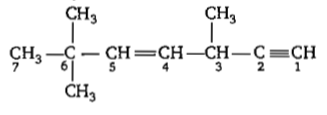The tendency of BF3, BCl3, and BBr3 to behave as Lewis acid decreases in the sequence

(a) BCl3 > BF3 > BBr3

(b) BBr3 > BCI3 > BF3

(c) BBr3 > BF3 > BCl3

(d) BF3 > BCl3 > BBr3

Concept Questions :-

Covalent Bonding
High Yielding Test Series + Question Bank - NEET 2020

Difficulty Level:

The state of hybridisation of C2, C3, C5 and C6 of the hydrocarbon,is in the following sequence
(a) sp, sp3, sp2 and sp3
(b) sp3, sp2, sp2 and sp
(c) sp, sp2, sp2 and sp3
(d) sp, sp2 sp3 and sp2

Concept Questions :-

Hybridisation
High Yielding Test Series + Question Bank - NEET 2020

Difficulty Level:

In which of the following molecules/ions BF3, ${\mathrm{NO}}_{2}^{-}$, ${\mathrm{NH}}_{2}^{-}$ and H2O, the central atom is sp2 hybridised?
(a) ${\mathrm{NO}}_{2}^{-}$ and ${\mathrm{NH}}_{2}^{-}$
(b) ${\mathrm{NH}}_{2}^{-}$ and H2O
(c) ${\mathrm{NO}}_{2}^{-}$ and H2O
(d) BF3, and ${\mathrm{NO}}_{2}^{-}$

Concept Questions :-

Hybridisation
High Yielding Test Series + Question Bank - NEET 2020

Difficulty Level:

What is the dominant intermolecular force on bond that must be overcome in converting liquid CH3OH to a gas?

(a) Hydrogen bonding

(b) Dipole-dipole interaction

(c) Covalent bonds

(d) London dispersion force

Concept Questions :-

Hydrogen Bonding
High Yielding Test Series + Question Bank - NEET 2020

Difficulty Level:

The angular shape of ozone molecule (O3) consists of

(a) 1 sigma and 2 pi bonds

(b) 2 sigma and 2 pi bonds

(c) 1 sigma and 1 pi bonds

(d) 2 sigma and 1 pi bonds

Concept Questions :-

Types of Bonding
High Yielding Test Series + Question Bank - NEET 2020

Difficulty Level:

The correct order of increasing bond angles in the following triatomic species is

(a) NO2- < NO2+ < NO2

(b) NO2- < NO2   < NO2+

(c) NO2< NO2   <NO2-

(d) NO2+ < NO2< NO2

Concept Questions :-

V.S.E.P.R
High Yielding Test Series + Question Bank - NEET 2020

Difficulty Level:

In the hydrocarbon

CH3-CH=CH-CH2-C$\equiv$CH

6      5     4   3      2    1

The state of hybridisation of carbons 1,3 and 5 are in the following sequence

(a) sp2, sp, sp3                       (b) sp, sp3, sp2

(c) sp, sp2, sp3                       (d) sp3, sp2, sp

Concept Questions :-

Hybridisation
High Yielding Test Series + Question Bank - NEET 2020

Difficulty Level:

In which of the following molecules are all the bonds not equal ?

(a) ClF3

(b) BF3

(c) AlF3

(d) NF3

Concept Questions :-

Hybridisation
High Yielding Test Series + Question Bank - NEET 2020

Difficulty Level:

The correct order of electronegativity of hybrid orbitals of carbon is:

(a) sp>sp2<sp3              (b) sp>sp2>sp3

(c) sp<sp2>sp3              (d) sp<sp2<sp3

Concept Questions :-

Hybridisation
High Yielding Test Series + Question Bank - NEET 2020

Difficulty Level:

Which of the following species has a linear shape?

(a) ${\mathrm{NO}}_{2}^{-}$          (b) SO2            (c) ${\mathrm{NO}}_{2}^{+}$              (d) O3

Concept Questions :-

Hybridisation